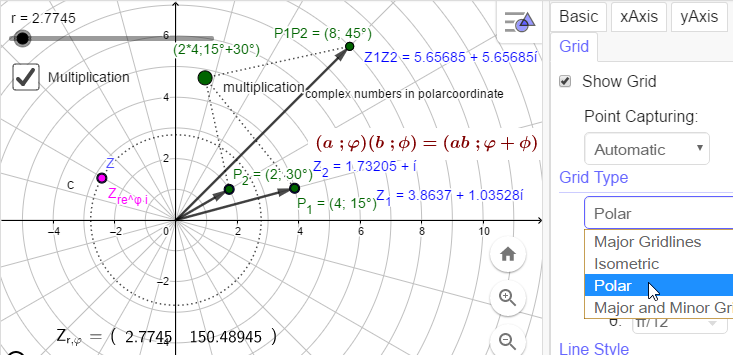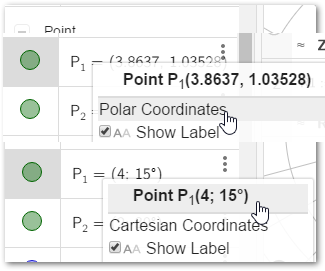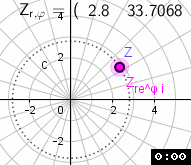# Visualizing Complex Numbers & Polynomials

Author:
hawe

## a complex story

Zi complex number x + i y, Zi cartesian-coordinates (x , y), Pi complex number in polar-coordinates (r ; φ) Multiplication Multiplication Z1*Z2 = Z1Z2 <===> P1*P2 = P1P2 Euler's Notation r eφ i P1P2 = 4 e15° i 2 e30° i = 4*2 e15° i + 30° i = 8 e45° iInput of polar coordinates (r ; φ) but ggb always gives back cartesian cordinates independent of the coordinates displayed: + Complex Number Z_{1} = 3.8637 + 1.03528ί + Point P_{1} = Z_{1}P1 =3.863703305156 + 1.03527618041 * ί x(P1)=3.863703305156 - noway to read/get r ; φ polar coordinates to use polar coordinates we have to calculate a conversion c=Circle((0, 0), r) Z=Point(c) Z_{re^φ i}=sqrt(x(Z)² + y(Z)²) ℯ^(atan(y(Z) / x(Z)) ί )calculating the angle of polar coordinates we have to take care about the quadrant of the tangens and a division by zero. so I have to discuss some cases to synchronize the angles given by the signs of trigonometric functions in each quadrant - define a function: pi(x,y):=If(x > 0 ∧ y ≥ 0, atand(y / x), x > 0 ∧ y < 0, atand(y / x) + 360°, x < 0, atand(y / x) + 180°, x ≟ 0 ∧ y > 0, 90°, x ≟ 0 ∧ y < 0, 3 / 2 * 180°, x ≟ 0 ∧ y ≟ 0, 0) ===> Z_{re^φ i}=sqrt(x(Z)² + y(Z)²) ℯ^(pi(x(Z), y(Z)) ί) or
 ﻿Input Point/Vector Z ﻿Input Complex Number x ﻿pϕ(x, y) = If(x ≠ 0, atan(y / x), y > 0, π / 2, 3 / 2 π) pr(x, y) = If(x ≠ 0, sgn(x), 1) sqrt(x² + y²) ===>pr(x(Z), y(Z)) ℯ^(pϕ(x(Z), y(Z)) ί) ﻿cϕ(x) =If(real(x) ≠ 0, atan(imaginary(x) / real(x)), If(imaginary(x) > 0, π / 2, 3 / 2 π)) cr(x) = If(real(x) ≠ 0, sgn(real(x)), 1) sqrt(real(x)² + imaginary(x)²) ===>cr(x) ℯ^(cϕ(x) ί)
the 2 points runing synchronous now - calculate correct angle φ !

## making classic app of

There is a problem (in AlgebraView ) when reading mixed lists (like ROOTS - has Complex Numbers and Numbers) with element therefore it is converted to Cartesian coordinates when processed in AlgebraView.